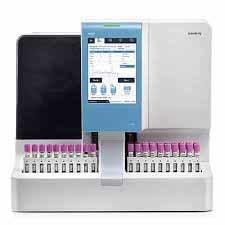# HPLC AnalyserHPLC Analyser
Product Code : KMS-544
Brand Name : KWALITY INDIA
Price And Quantity
• Minimum Order Quantity
• 150 Piece
• Price
• 10000 INR
Product Specifications
• Dimension(L*W*H)
• 70 x 60 x 70 cm Millimeter (mm)
Product Description
##### Column Oven

The column over compartment work from -5*C Ambient to 76*C.

The Temperature stability +-0.15*C.

It support 3 nos of 30 cm long column and offer 2 different heating zones.

##### HPLC Detector

The DPA/PDA wavelength of 190 cm to 950 cm with accuracy  of +-1 nm and for better sensitivity the no of Hz.

The drift within 0.9 mAU at 254 nm.

Other Features

C-18 column, 25 cm, 4.6 mm ID 0.5 practical size.

The tool kit, starter lit quoted for routine running and maintenance of HPLC system.

##### Technical Specification

 Test Set points/Range Acceptance Criteria Flow rate and precision Flow rate 1 = 0.5 ml/min Flow rate 2 = 1.0 ml/min Flow rate 3 = 5.0 ml/min Accuracy <=5% from set point Precision <=0.50% RSD Column compartment Temperature Accuracy Temperature 1 = 30.0*C Temperature 2 = 45.0*C Temperature 3 = 60.0*C +-2*C Sampler Cooler Accuracy Temperature 1 = 4*C Difference from set point >=2*C, <=5*C Detector Accuracy (UV-VIS) Wavelength 1 =205 nm (Max) Wavelength 2 = 273 nm (Max) +-2nm Detector Accuracy (FLD) Wavelength 1 =350 nm (Max) Wavelength 2 = 397 nm (Max) +-3nm Gradient composition Accuracy for isocratic and quaternary pumps 20% Steps Peak 2: 20+-2%nm Peak 3: 20+-2%nm Gradient composition Accuracy for Binary pumps 25% and 75% Steps Peak 2: 25+-2%nm Peak 3: 75+-2%nm Injector Accuracy Injection Injection Volume 1 = 50 ul +-2 ul Injector Precision (UV-VIS) Injection Volume 1 = 20 ul Area and Retention time RSD <= 1.0% Injector Precision (RID) Injection Volume 1 = 20 ul Area RSD <= 3.0% Retention time RSD <= 2.0% Carryover Injection Volume 1 = 20 ul <=0.2% Carryover (FLC/RID) Injection Volume 1 = 5 ul <=10.0% Injection/Detector Linearity Injection Volume 1 = 5 ul Injection Volume 2 = 10 ul Injection Volume 3 = 25 ul Injection Volume 4 = 50 ul Injection Volume 5 = 100 ul R2 <=0.999 For RID: R2 >=0.999 RID Precision Injection Injection Volume 1 = 20 ul Retention Time: %RSD; <=2.0% Peak Response: <=3.0% FLD Precision Injection Volume 1 = 50 ul <=2.0%

##### Specifications

 Cat No. KMS-544 Interior Dimensions 200 x 120 x 70 cm (LxHxW) Power 60 Hz Exhaust Volume 270 cubic feet/min. Blower Dimension 35 x 35 x 40 mm (LxHxW) Filter Both chemical fumes and dust particles Voltage 220/240 V